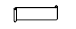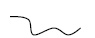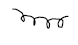# CBSE Class 5 Mathematics Geometry MCQs

## MCQ for Class 5 Mathematics Geometry

Class 5 Mathematics students should refer to the following multiple-choice questions with answers for Geometry in standard 5. These MCQ questions with answers for Grade 5 Mathematics will come in exams and help you to score good marks

### Geometry MCQ Questions Class 5 Mathematics with Answers

Question: A (n) ___________ triangle has all slides of equal length.

a) equilateral

b) right angle

c) scalene

Question: A nanogon has ___________ sides

a) 9

b) 7

c) 6

Question: A rhombus is always a square.

a) False

b) True

c) Both

Question: A line has ___________ end points

a) no

b) one

c) two

Question: The longest chord of a circle is ___________.

a) diameter

b) circumference

Question: A quadrilaterals has ___________ diagonals.

a) two

b) four

c) three

Question: An angle of 89° is ___________ angle.

a) acute

b) right

c) obtuse

Question: An angle of 95° is ___________ angle.

a) obtuse

b) refle

c) Right

Question: A line segment has ___________ end point (s).

a) two

b) no

c) one

Question: A ray has ___________ end point (s).

a) one

b) two

c) no

Question: An octagon has ___________ sides.

a) 8

b) 6

c) 4

Question: A hexagon has ___________ sides.

a) 6

b) 5

c) 3

Question: Interesting lines are always, perpendicular.

a) False

b) True

Question: A line has fixed length.

a) False

b) True

Question: Half of circle is called diameter.

a) Semicircle

b) Diagonal

c) True

Question: A triangle has its three side equal is

a) equilateral

b) isoscales

c) scalene

Question: A triangle with one right angle is called ___________ triangle.

a) right

b) obtuse

c) scalene

Question: From a given point, infinite number of rays can be drawn.

a) True

b) False

Question: A pentagon has ___________ sides.

a) 5

b) 8

c) 7

Question: An angle of 180° is called ___________ angle.

a) straight

b) acute

c) reflex

Question: Which is a closed figure.

a)b)c)Question: A square is a rectangle

a) True

b) False

Question: In a rectangle opposite sides are different in length.

a) False

b) True

Question: A triangle has ___________ vertices.

a) 3

b) 2

c) 1